# 前端必看的js对象深拷贝方法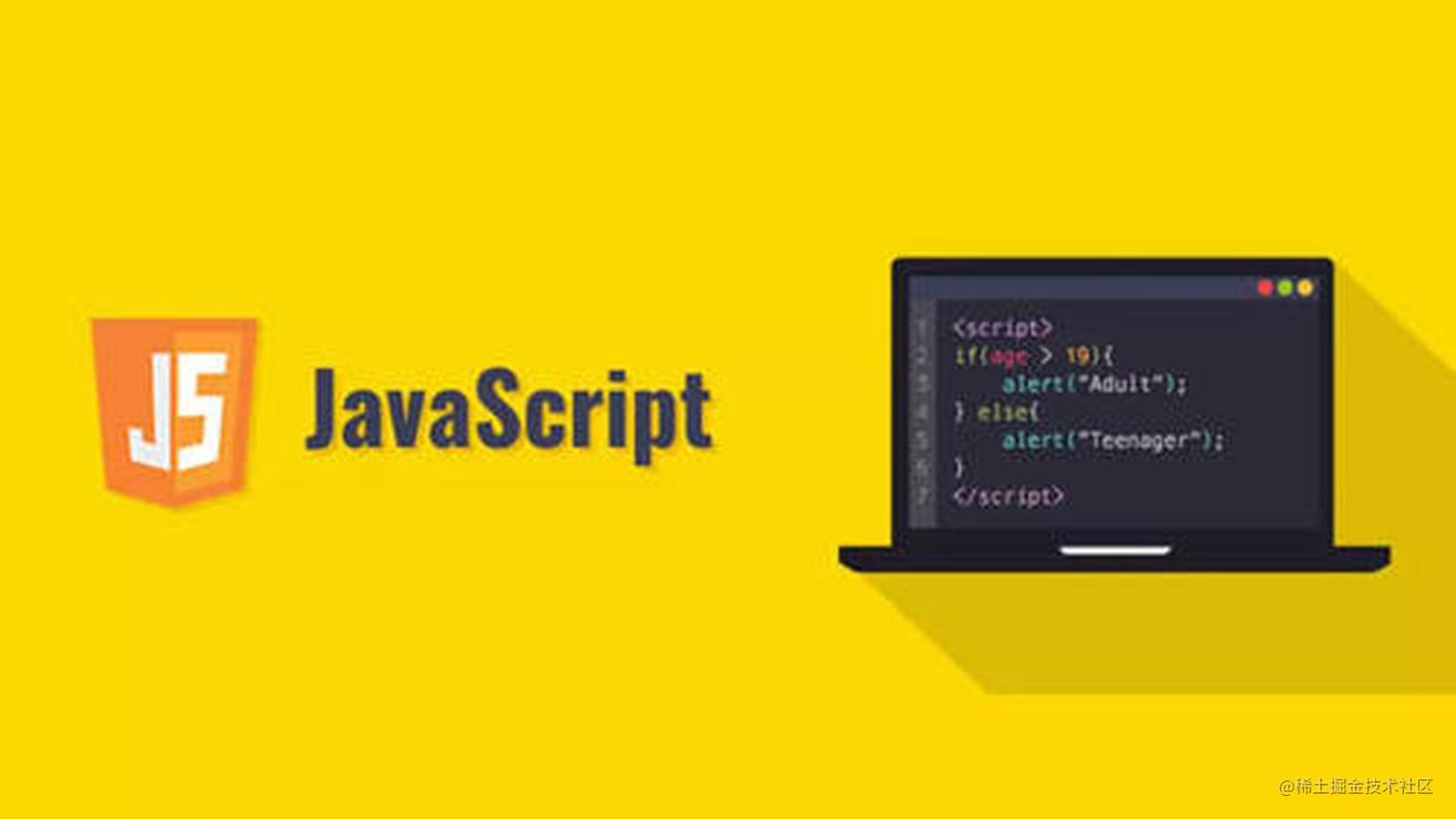## 前言

首先js的数据值按照类型主要分为两大类，基本数据类型引用数据类型。基本数据类型包括Undefined、Null、Number、String、Boolean、Symbol；引用数据类型则为Object,那些Array、Set、Map数据也属于Object。

``````// 基本数据类型赋值
var a = 'aaa';
var b = a;
console.log(a);  // 'aaa'
console.log(b);  // 'aaa'
b = 'bbb';
console.log(a);  // 'aaa'
console.log(b);  // 'bbb'

// 引用数据类型赋值
var a = {    name: '张三'};
var b = a;
console.log(a);  // {name: "张三"}
console.log(b);  // {name: "张三"}
b.name = '李四';
b.age = 18;
console.log(a);  // {name: "李四", age: 18}
console.log(b);  // {name: "李四", age: 18}复制代码``````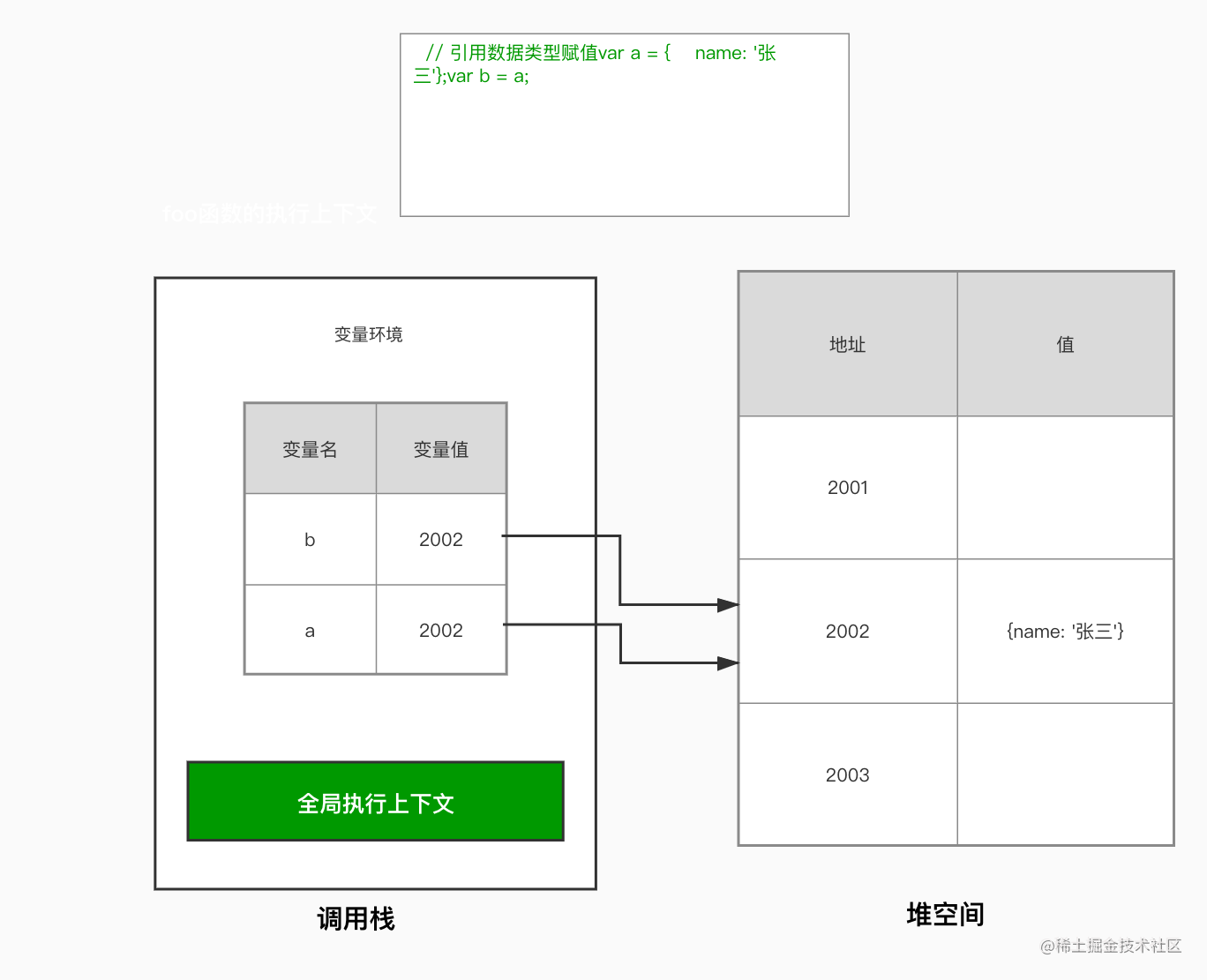## 深拷贝方法

### 1. JSON.parse(   JSON.stringify()   ) 序列化和反序列

``````// 测试数据
var test = {  name: "test"};
var data = {  a: "123",
b: 123,
c: true,
d: [43, 2],
e: undefined,
f: null,
g: function() {    console.log("g");  },
h: new Set([3, 2, null]),
i: Symbol("fsd"),
j: test,
k: new Map([    ["name", "张三"],    ["title", "Author"]  ])
};

JSON.stringify(data) 复制代码``````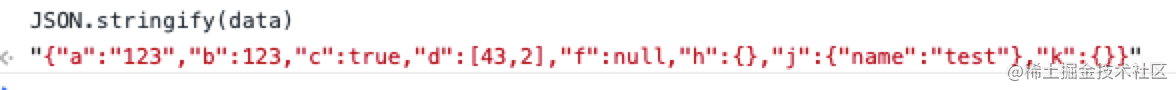``````// 测试数据
var data = {
name: 'foo',
child: null,
}
data.child = data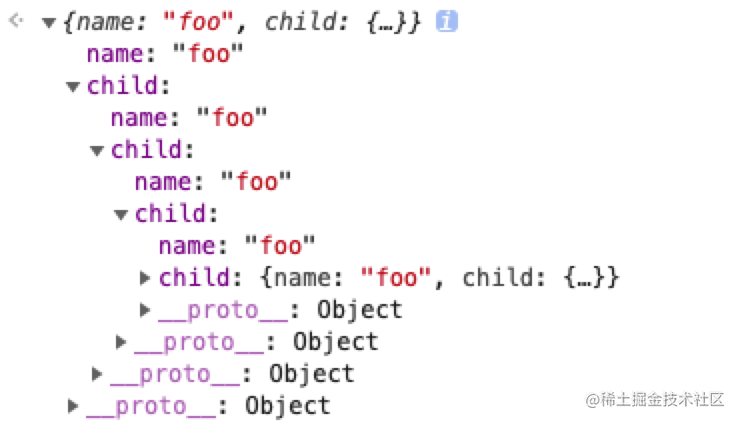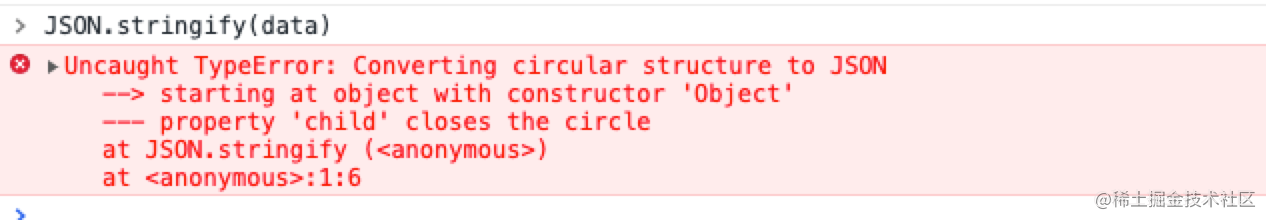``````// 测试数据
var test = {  name: "test"};
var data = {  a: "123",
b: 123,
c: true,
d: [43, 2],
e: test,
f: {
name: '张三',
age: 18,
likes: {
ball: ['足球','篮球']
}
}
};
JSON.stringify(data) 复制代码``````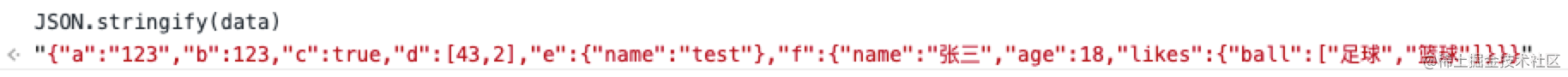``````function deepCopy(obj){
if(typeof obj === 'function'){
throw new TypeError('请传入正确的数据类型格式')
}
try {
let data = JSON.stringify(obj)
let newData = JSON.parse(data)
return newData
} catch(e) {
console.log(e)
}
}复制代码``````

### 2. Object.assign(target, source1, source2)

es6新增的方法，可用于对象合并，将源对象的所有可枚举属性，复制到目标对象上。

``````var data = {
a: "123",
b: 123,
c: true,
d: [43, 2],
e: undefined,
f: null,
g: function() {    console.log("g");  },
h: new Set([3, 2, null]),
i: Symbol("fsd"),
k: new Map([    ["name", "张三"],    ["title", "Author"]  ])
};

var newData = Object.assign({},data)
console.log(newData)  复制代码``````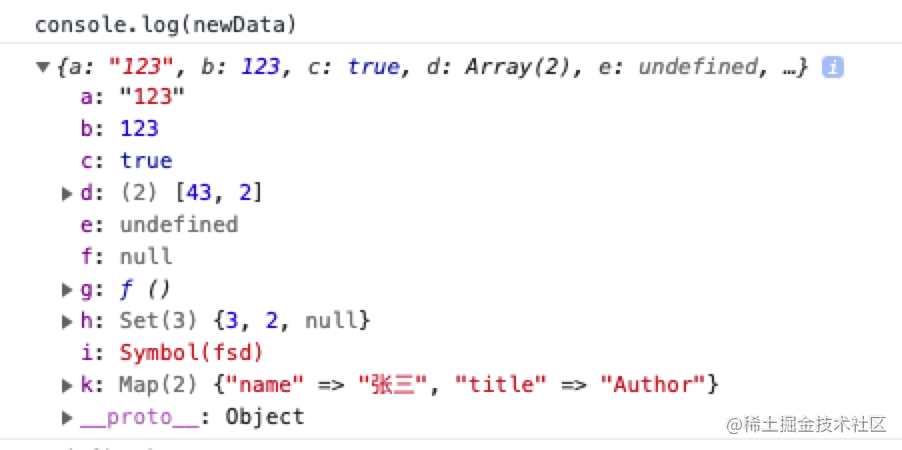``````var test = {  name: '张三' }
var data = {
a: 123,
b: test
}
var newData = Object.assign({},data)
console.log(newData)
// {  a: 123,  b: {    name: '张三'  }}
test.age = 18
console.log(newData)
// {  a: 123,  b: {    name: '张三',   age: 18  }}复制代码``````

### 3. 迭代递归方法

``````function deepCopy(data) {
if(typeof data !== 'object' || data === null){
throw new TypeError('传入参数不是对象')
}
let newData = {};
const dataKeys = Object.keys(data);
dataKeys.forEach(value => {
const currentDataValue = data[value];
// 基本数据类型的值和函数直接赋值拷贝
if (typeof currentDataValue !== "object" || currentDataValue === null) {
newData[value] = currentDataValue;
} else if (Array.isArray(currentDataValue)) {
// 实现数组的深拷贝
newData[value] = [...currentDataValue];
} else if (currentDataValue instanceof Set) {
// 实现set数据的深拷贝
newData[value] = new Set([...currentDataValue]);
} else if (currentDataValue instanceof Map) {
// 实现map数据的深拷贝
newData[value] = new Map([...currentDataValue]);
} else {
// 普通对象则递归赋值
newData[value] = deepCopy(currentDataValue);
}
});
return newData;
}复制代码``````

``````// 测试数据项
var data = {
age: 18,
name: "liuruchao",
education: ["小学", "初中", "高中", "大学", undefined, null],
likesFood: new Set(["fish", "banana"]),
friends: [
{ name: "summer",  sex: "woman"},
{ name: "daWen",   sex: "woman"},
{ name: "yang",    sex: "man" }  ],
work: {
time: "2019",
project: { name: "test",obtain: ["css", "html", "js"]}
},
play: function() {    console.log("玩滑板");  }
}复制代码``````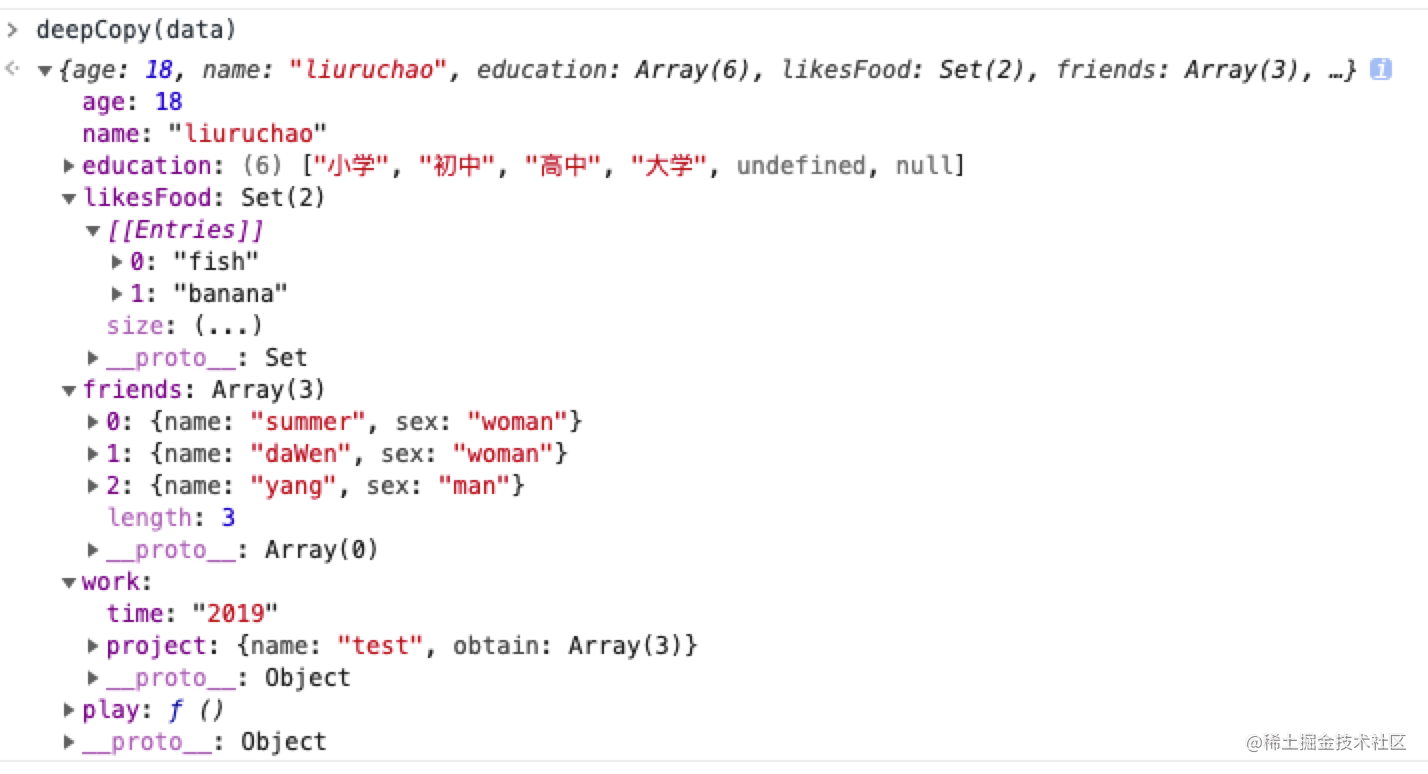``````// 测试数据
var data = {
name: 'foo',
child: null,
}
data.child = data

deepCopy(data)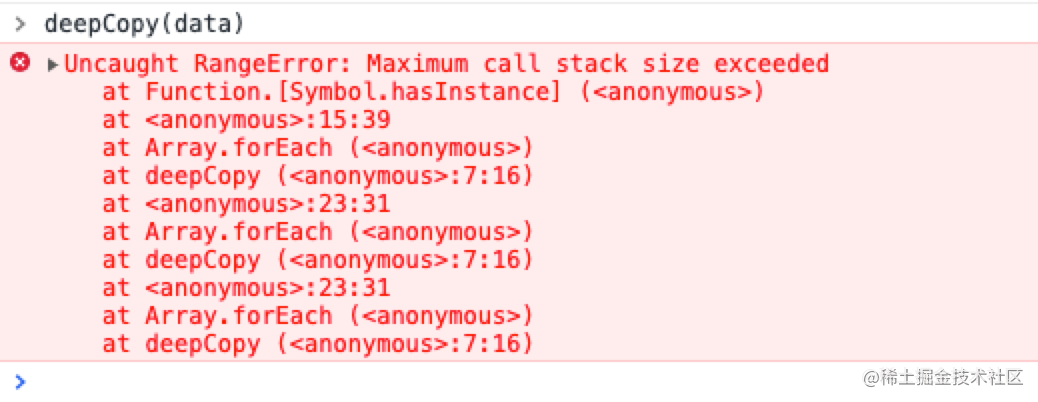### 4. 迭代递归方法（解决闭环问题）

``````function deepCopy(data, hash = new WeakMap()) {
if(typeof data !== 'object' || data === null){
throw new TypeError('传入参数不是对象')
}
// 判断传入的待拷贝对象的引用是否存在于hash中
if(hash.has(data)) {
return hash.get(data)
}
let newData = {};
const dataKeys = Object.keys(data);
dataKeys.forEach(value => {
const currentDataValue = data[value];
// 基本数据类型的值和函数直接赋值拷贝
if (typeof currentDataValue !== "object" || currentDataValue === null) {
newData[value] = currentDataValue;
} else if (Array.isArray(currentDataValue)) {
// 实现数组的深拷贝
newData[value] = [...currentDataValue];
} else if (currentDataValue instanceof Set) {
// 实现set数据的深拷贝
newData[value] = new Set([...currentDataValue]);
} else if (currentDataValue instanceof Map) {
// 实现map数据的深拷贝
newData[value] = new Map([...currentDataValue]);
} else {
// 将这个待拷贝对象的引用存于hash中
hash.set(data,data)
// 普通对象则递归赋值
newData[value] = deepCopy(currentDataValue, hash);
}
});
return newData;
}复制代码``````

1. 首次调用时，weakMap为空，不会走上面那个if(hash.has())语句，如果待拷贝对象中有属性也为对象时，则将该待拷贝对象存入weakMap中，此时的健值和健名都是对该待拷贝对象的引用
2. 然后递归调用该函数
3. 再次进入该函数，传入了上一个待拷贝对象的对象属性的引用和存储了上一个待拷贝对象引用的weakMap，因为如果是循环引用产生的闭环，那么这两个引用是指向相同的对象的，因此会进入if(hash.has())语句内，然后return，退出函数，所以不会一直递归进栈，以此防止栈溢出。